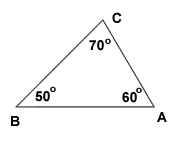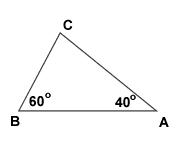# How are the three angles related?

Just like regular numbers, angles can be added to obtain a sum, perhaps for the purpose of determining the measure of an unknown angle. Sometimes we can determine a missing angle because we know that the sum must be a certain value. Remember -- the sum of the degree measures of angles in any triangle equals 180 degrees. Below is a picture of triangle ABC, where angle A = 60 degrees, angle B = 50 degrees and angle C = 70 degrees.If we add all three angles in any triangle we get 180 degrees. So, the measure of angle A + angle B + angle C = 180 degrees. This is true for any triangle in the world of geometry. We can use this idea to find the measure of angle(s) where the degree measure is missing or not given.

### Finding a Missing Angle

In triangle ABC below, angle A = 40 degrees and angle B = 60 degrees. What is the measure of angle C?We know that the sum of the measures of any triangle is 180 degrees. Using the fact that angle A + angle B + angle C = 180 degrees, we can find the measure of angle C.

angle A = 40
angle B = 60
angle C = we don't know.

To find angle C, we simply plug into the formula above and solve for C.

A + B + C = 180
C = 180 - A - B
C = 180 - 40 - 60
C = 80

To check if 80 degrees is correct, let's add all three angle measures. If we get 180 degrees, then our answer for angle C is right.

Here we go:

40 + 60 + 80 = 180
180 = 180...It checks!

You don't always have to plug in those values to the equation and solve. Once you're comfortable with this sort of problem you'll be able to say "okay, 40 + 60 =100, so the other angle has to be 80!" and it's much quicker.

### Equilateral Triangles

If a triangle is equilateral, what is the degree measure of each of its angles?

Remember, all sides of an equilateral triangle have equal measure. They also, as you'll learn, have equal angles! Let x = the degree measure of each angle. Triangles have three angles and so we will add x THREE times.

We have this:

x + x + x = 180
3x = 180
x = 60

Makes sense, right? If all the angles are equal, and they add up to 180, then it has to be 60 degrees!

### A Ratio of Angles

The degree measures of the angles of a triangle are in the ratio 4 : 5 : 9.
What is the degree measure of the SMALLEST angle of the triangle?

Notice that the smallest angle is represented by the smallest number in the ratio given. The smallest number given is 4, right? Since this is a ratio, we have to multiply all those values (4,5,9) by some common factor to get the actual angles. (For example, 60 and 80 are in a 3:4 ratio with a factor of 20)

Let 4x = the measure of the smallest angle of the triangle. We can now say that 5x and 9x = the degree measures of the remaining angles of the triangle. We simply add 4x + 5x + 9x, equated the sum to 180 degrees and solve for x. After finding x, we plug the value of x into 4x, and simplify to find the measure of the smallest angle of the triangle at hand.

4x + 5x + 9x = 180
9x + 9x = 180
18x = 180
x = 180/18
x = 10

We found the value of x but it does NOT mean we are done.
To find the measure of the smallest angle of the triangle, we multiply 4 times 10. So, 4 x 10 = 40.